Download the eSaral app and start learning from Kota's top IITians and doctors.

# Types & Effects of Electric Current | Current Density

In electrostatics, we studied charges at rest. In real life the most important application without which we can't think of our lives i.e. electricity is produced by charges in motion. Charges in motion constitute electric current. Here we will see the basic things like Unit of Electric Current, Types of Electric Current, Effects of Electric Current and Current density. Let's start:

## Introduction to Electric Current

Electricity, phenomenon associated with stationary or moving electric charges. Electric charge is a fundamental property of matter and is borne by elementary particles. In electricity the particle involved is the electron, which carries a charge designated, by convention, as negative. Thus, the various manifestations of electricity are the result of the accumulation or motion of numbers of electrons. [source] Electric Current is defined as rate of flow of charge through any cross-section. If $\Delta q$ charge passes through a cross-section in time $\Delta {\rm{t}}$ then average current ${{\rm{I}}_{{\rm{av}}}} = {{\Delta {\rm{q}}} \over {\Delta {\rm{t}}}}\quad$and $\quad$ instantaneous current ${\rm{I}} = \mathop {\lim }\limits_{\Delta {\rm{t}} \to 0} {{\Delta {\rm{q}}} \over {\Delta {\rm{t}}}} = {{{\rm{dq}}} \over {{\rm{dt}}}}$

## Unit of Electric Current

Current is a fundamental quantity with dimension $\mathrm{M}^{\circ} \mathrm{L}^{\circ} \mathrm{T}^{\circ} \mathrm{A}^{1}$ Current is a scalar quantity with its SI unit Ampere. Ampere: The current through a conductor is said to be one ampere if one coulomb of charge is flowing per second through a cross-section of wire. CGS unit of current is Biot (Bi) 1 ampere $= {{1{\rm{ coulomb }}} \over {{\rm{ sec ond }}}} = {{(1/10){\rm{ emu of charge }}} \over {{\rm{ sec ond }}}} = {1 \over {10}}$ Biot

### Important Points

1. The conventional direction of current is the direction of flow of positive charge or applied field. It is opposite to direction of flow of negatively charged electrons. [caption id="attachment_4538" align="aligncenter" width="681"]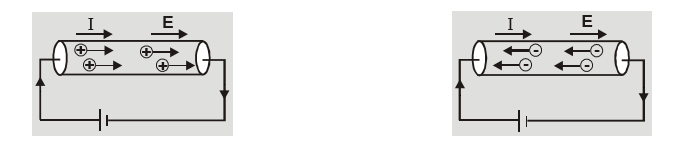Direction of Electric Current[/caption]
2. The current may be constituted by motion of different type of charge carries in different situations. [caption id="attachment_4539" align="aligncenter" width="561"]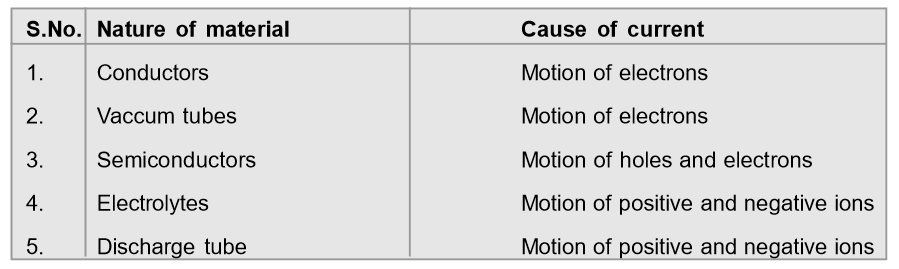Different charge carriers in different type of materials[/caption]
3. If n electrons pass through a point in a conductor in time $t$ then current through the conductor is ${\rm{I}} = {{\rm{q}} \over {\rm{t}}} = {{{\rm{ne}}} \over {\rm{t}}}$. Number of electrons flowing through conductor in ${\rm{t}}$ second is${\rm{n}} = {{{\rm{I}} \times {\rm{t}}} \over {\rm{e}}}$. For 1 ampere of current ${\rm{n}} = {1 \over {1.6 \times {{10}^{ - 19}}}} = 6.25 \times {10^{18}}$ electrons/second
4. The conductor remains uncharged when current flows through it because the charge entering at one end per second is equal to charge leaving the other end per second.
5. For a given conductor current does not change with change in its cross-section because current is simply rate of flow of charge. [caption id="attachment_4540" align="aligncenter" width="227"]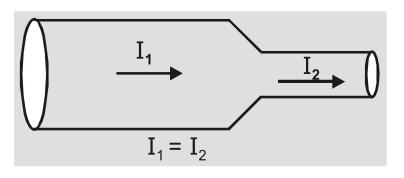Electric Current doesn't depend upon area of cross-section[/caption]
6. If n particles each having a charge q pass per second per unit area then current associated with cross-sectional area A is ${\rm{I}} = {{\Delta {\rm{q}}} \over {\Delta {\rm{t}}}} = {\rm{nqA}}$
7. If there are n particles per unit volume each having a charge q and moving with velocity v then current through cross-sectional area A is ${\rm{I}} = {{\Delta {\rm{q}}} \over {\Delta {\rm{t}}}} = {\rm{nqvA}}$
8. If a charge q is moving in a circle of radius r with speed v then its time period is T = 2πr/v. The equivalent current ${\rm{I}} = {{\rm{q}} \over {\rm{T}}} = {{{\rm{qv}}} \over {2\pi {\rm{r}}}}$

## Current Density

This is defined as current flowing per unit area held normal to direction of flow of current. Current density $J = {I \over A} = {{nq} \over {At}}$ and current $I = \int {\vec J} \cdot \overrightarrow {da}$ The direction of current density $\vec \jmath$ is same as that of electric field $\vec E.$ J is a vector with unit ${\rm{amp}}/{{\rm{m}}^2}$and dimension $\mathrm{M}^{\circ} \mathrm{L}^{\circ} \mathrm{T}^{\circ} \mathrm{A}^{1}$

## Effects of Electric Current

### (a) Heating Effects of Electric Current

The phenomenon of heating of a conductor by the flow of an electric current through it is called heating effect of electric current.

### (b) Magnetic Effects of Electric Current

The phenomenon of production of a magnetic field in space around a current carrying conductor is called magnetic effect of electric current.

### (c) Chemical Effects of Electric Current

The phenomenon of decomposition of an electrolyte into ions on passing an electric current through it is called chemical effect of electric current. [caption id="attachment_4541" align="aligncenter" width="558"]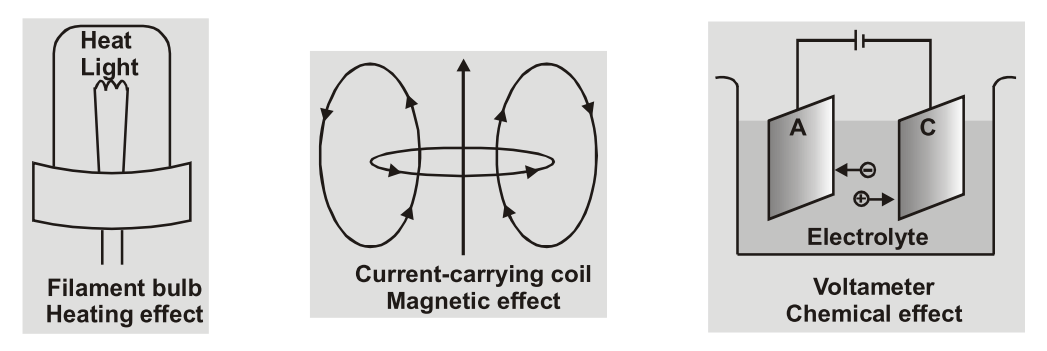Effects of Electric Current[/caption]

## Types of Electric Current

### (a) Direct Current

The current whose magnitude and direction does not vary with time is called direct current (dc). The various sources are cells, battery, dc dynamo etc. [caption id="attachment_4542" align="aligncenter" width="219"]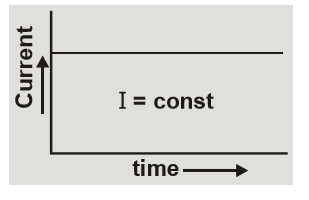Direct Current[/caption]

### (b) Alternating Current

The current whose magnitude continuously changes with time and periodically changes its direction is called alternating current. It has constant amplitude and has alternate positive and negative halves. It is produced by ac dynamo. [caption id="attachment_4543" align="aligncenter" width="314"]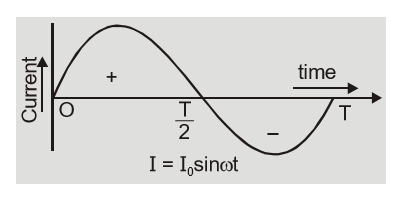Alternating Current[/caption]     Also Read: